# Polyrhons of Order 1..4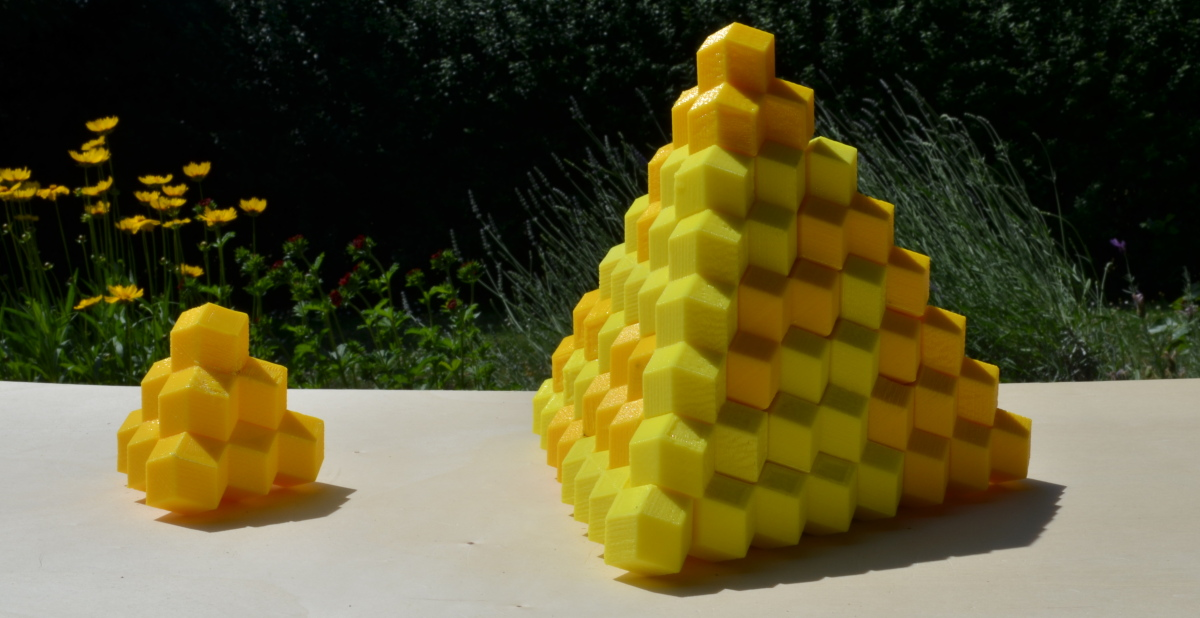Above is a construction with all polyrhons with 1 to 4 rhombic dodecahedrons. Beside the tetrarhons all smaller pieces are used.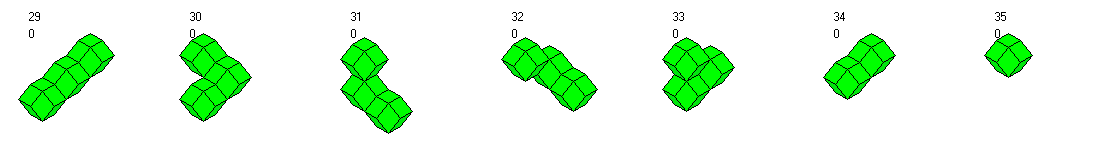Together we have 130 rhombic dodecahedrons, 120 for the large tetrahedron and 10 for the small one. Since there are not many pieces suitable for the small tetrahedron we must start with this figure. This is a small puzzle for its own. Given the tetrarhon number 12, the straight trirhon and the triangle trirhon it may take you one or two minutes to find a solution.

Roofs with bottoms of size 10x5 and 14x4 are also possible. Click the pictures to see the layers.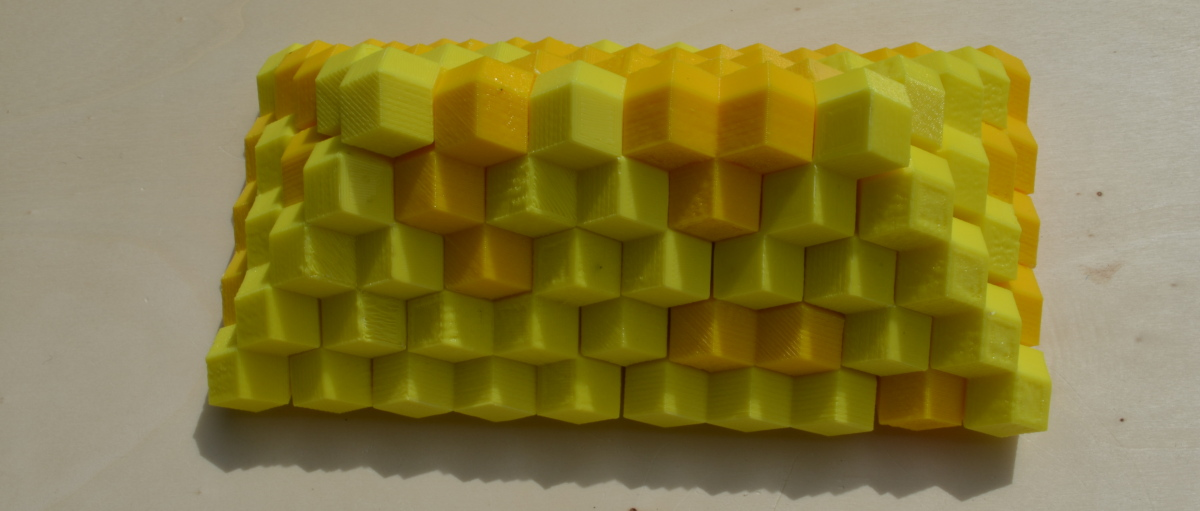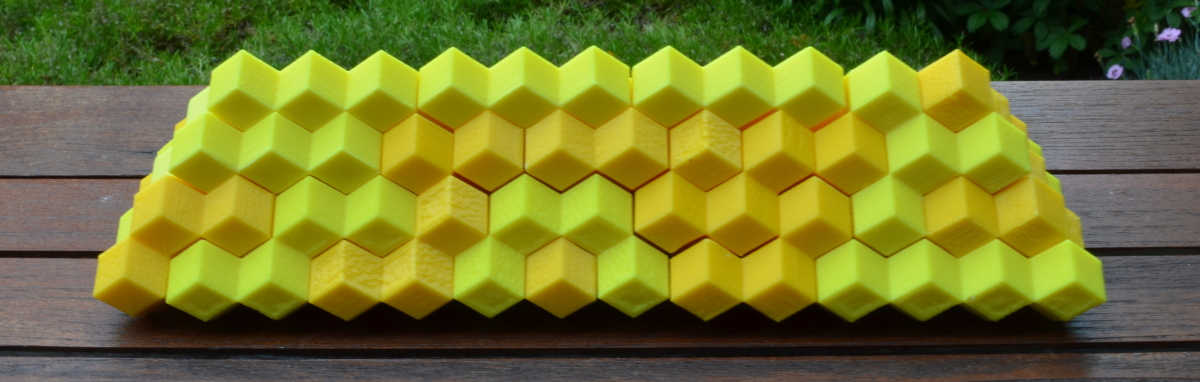Similar constructions with rhombs at the bottom may be possible, too.

As with the tetrarhons we can try to stack 2n-1 layers of alternating size. To use the whole set of polyrhons of order 1..4 we can look for integer solutions for the following equations.
• (a x b)*n + (a+1)x(b+1)*(n-1)=130, => n=10, a=1, b=4, a,b interchangeable
• (a x b)*n + (a-1)x(b-1)*(n-1)=130, => n=6, a=5, b=3, or n=5, a=6, b=3 or n=3, a=6, b=5, a,b interchangeable
• (a x b)*n + (a-1)x(b+1)*(n-1)=130, => n=5, a=9, b=2 or n=2, a=9,b=5

To identify the different constructions it seems to be better to assume that corners are at the bottom. If you turn the blocks to get the rhombs on the bottom, the corners are at the left and right vertical sides and the physical constructions are often more stable.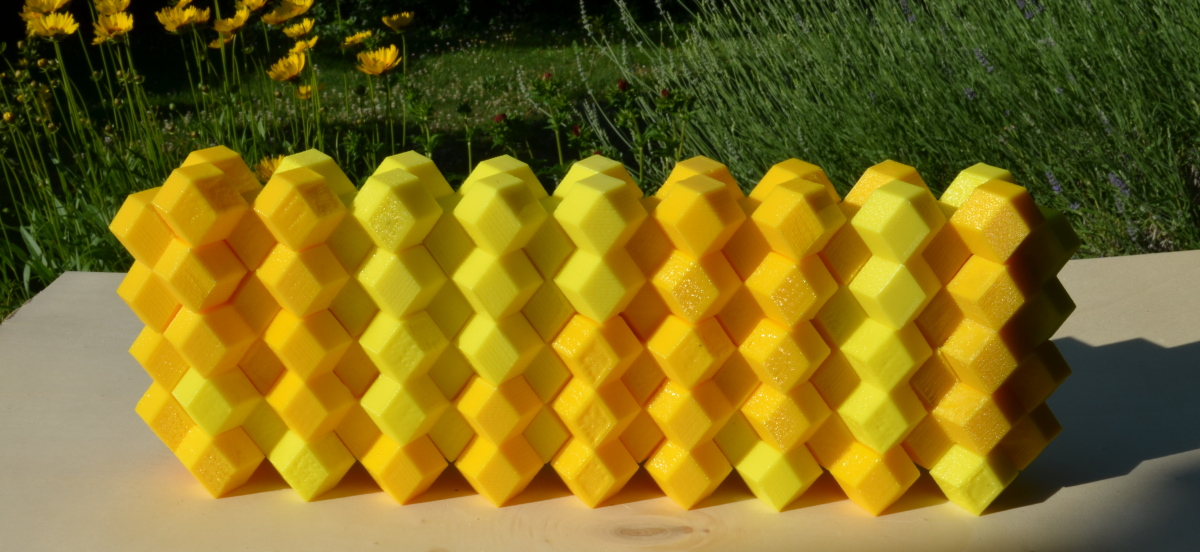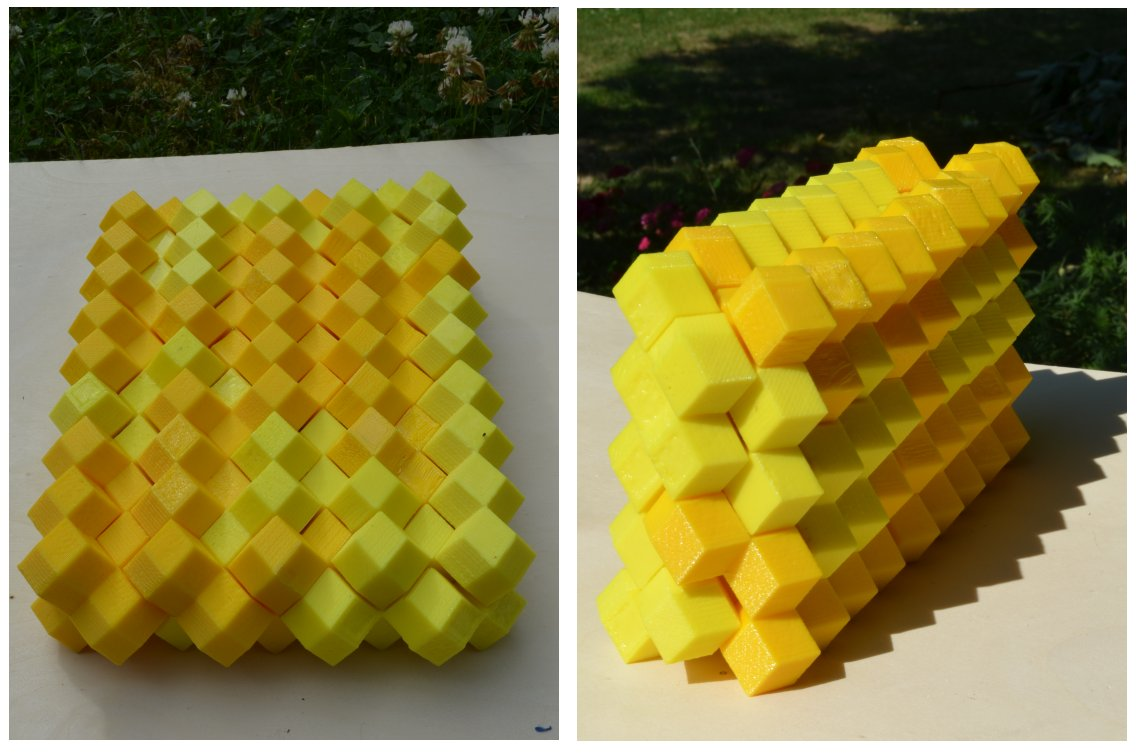Back
Home